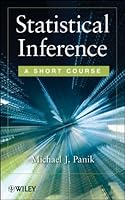# Statistical Inference: A Short Course1 Reviews
2012-07-03
400 pages

## Book Description

A concise, easily accessible introduction to descriptive and inferential techniques

Statistical Inference: A Short Course offers a concise presentation of the essentials of basic for readers seeking to acquire a working knowledge of statistical concepts, measures, and procedures.

The author conducts tests on the assumption of randomness and normality, provides nonparametric methods when parametric approaches might not work. The book also explores how to determine a confidence interval for a population median while also providing coverage of ratio estimation, randomness, and causality. To ensure a thorough understanding of all key concepts, Statistical Inference provides numerous examples and solutions along with complete and precise answers to many fundamental questions, including:

• How do we determine that a given dataset is actually a random sample?
• With what level of precision and reliability can a population sample be estimated?
• How are probabilities determined and are they the same thing as odds?
• How can we predict the level of one variable from that of another?
• What is the strength of the relationship between two variables?

The book is organized to present fundamental statistical concepts first, with later chapters exploring more advanced topics and additional statistical tests such as Distributional Hypotheses, Multinomial Chi-Square Statistics, and the Chi-Square . Each chapter includes appendices and exercises, allowing readers to test their comprehension of the presented material.

Statistical Inference: A Short Course is an excellent book for courses on probability, statistics, and statistical inference at the upper-undergraduate and graduate levels. The book also serves as a valuable reference for researchers and practitioners who would like to develop further insights into essential statistical tools.

1 The Nature of Statistics 1
2 Analyzing Quantitative Data 9
3 Descriptive Characteristics of Quantitative Data 22
4 Essentials of Probability 61
5 Discrete Probability Distributions and Their Properties 81
6 The Normal Distribution 101
7 Simple Random Sampling and the Sampling Distribution of the Mean 122
8 Confidence Interval Estimation of m 152
9 The Sampling Distribution of a Proportion and its Confidence Interval Estimation 170
10 Statistical Hypotheses 184
11 Comparing Two Population Means and Two Population Proportions 217
12 Bivariate Regression and Correlation 253
13 An Assortment of Additional Statistical Tests 295

## Book Details

• Title: Statistical Inference: A Short Course
• Author:
• Length: 400 pages
• Edition: 1
• Language: English
• Publisher:
• Publication Date: 2012-07-03
• ISBN-10: 1118229401
• ISBN-13: 9781118229408